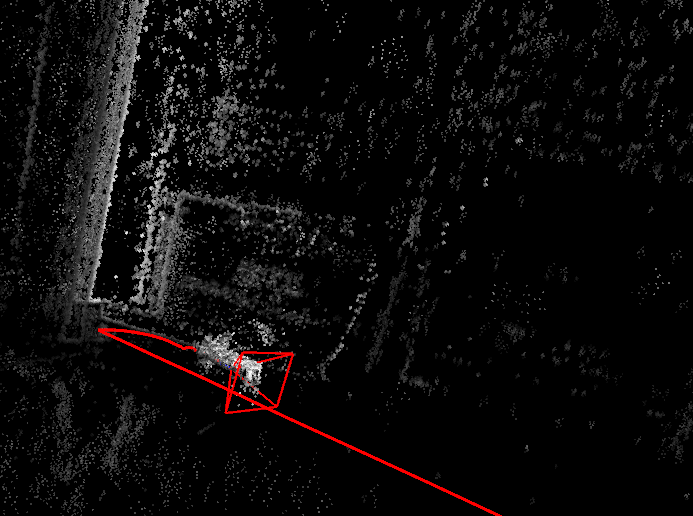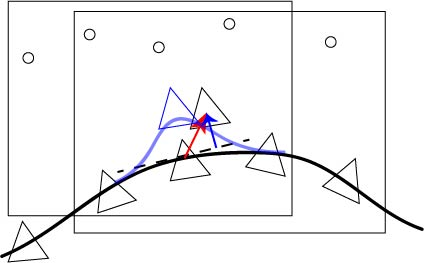# DSO零空间与尺度漂移

2023-09-24 13 0

DSO作为一种单目视觉里程计，观其代码用的也不是Sim3优化，那么它是如何保持零空间的稳定性的呢？这块的代码其实看得不是很懂，大致记录一下自己的理解。

### 零空间

H x = 0 (1) \mathbf H \mathbf x=\mathbf 0 \tag{1}

H x 1 = b (2) \mathbf H \mathbf x_1=\mathbf b \tag{2}
H x 2 = b (3) \mathbf H \mathbf x_2=\mathbf b \tag{3}

H ( x 2 − x 1 ) = 0 (4) \mathbf H (\mathbf x_2-\mathbf x_1)=\mathbf 0 \tag{4}
Δ x = x 2 − x 1 \Delta \mathbf x=\mathbf x_2-\mathbf x_1 ，这里的 Δ x \Delta \mathbf x 就是状态变量在零空间中的游荡量，表现在地图中可能是地图的尺度突然缩小，相机移动量也对应缩小，同时不会对能量函数造成影响，如下图1所示：### 怎么处理

DSO采用将增量正交化的方式来缓解这种情况，相应代码在函数EnergyFunctional::orthogonalize中。如图２所示，三角形代表相机位姿，黑色实现是相机轨迹，矩形框代表滑动窗口，圆点表示点，虚线表示途中第三帧相机位姿的零空间，红色箭头表示高斯牛顿法求解增量方程得到的解x，蓝色箭头表示最后求解出的x和零空间正交的分量。### 来看代码

VecX x;
x = SVecI.asDiagonal() * HFinalScaled.ldlt().solve(SVecI.asDiagonal() * bFinal_top);


orthogonalize(&x, 0);


// decide to which nullspaces to orthogonalize.
std::vector<VecX> ns;
ns.insert(ns.end(), lastNullspaces_pose.begin(), lastNullspaces_pose.end());
ns.insert(ns.end(), lastNullspaces_scale.begin(), lastNullspaces_scale.end());// make Nullspaces matrix
MatXX N(ns.rows(), ns.size());
for(unsigned int i=0;i<ns.size();i++)N.col(i) = ns[i].normalized();


// scale change
SE3 w2c_leftEps_P_x0 = (get_worldToCam_evalPT());
w2c_leftEps_P_x0.translation() *= 1.00001;
w2c_leftEps_P_x0 = w2c_leftEps_P_x0 * get_worldToCam_evalPT().inverse();
SE3 w2c_leftEps_M_x0 = (get_worldToCam_evalPT());
w2c_leftEps_M_x0.translation() /= 1.00001;
w2c_leftEps_M_x0 = w2c_leftEps_M_x0 * get_worldToCam_evalPT().inverse();
nullspaces_scale = (w2c_leftEps_P_x0.log() - w2c_leftEps_M_x0.log())/(2e-3);


for(int i=0;i<6;i++)
{Vec6 eps; eps.setZero(); eps[i] = 1e-3;SE3 EepsP = Sophus::SE3::exp(eps);SE3 EepsM = Sophus::SE3::exp(-eps);SE3 w2c_leftEps_P_x0 = (get_worldToCam_evalPT() * EepsP) * get_worldToCam_evalPT().inverse();SE3 w2c_leftEps_M_x0 = (get_worldToCam_evalPT() * EepsM) * get_worldToCam_evalPT().inverse();nullspaces_pose.col(i) = (w2c_leftEps_P_x0.log() - w2c_leftEps_M_x0.log())/(2e-3);
}


N Δ k = x (5) \mathbf N \Delta \mathbf k = \mathbf x \tag{5}

k 0 = ( N T N ) − 1 N T x (6) \mathbf k_0 = (\mathbf N^T\mathbf N)^{-1}\mathbf N^T\mathbf x \tag{6}

x ← x − N N † x (7) \mathbf x \gets \mathbf x-\mathbf N\mathbf N^{\dagger}\mathbf x \tag{7}

// compute Npi := N * (N' * N)^-1 = pseudo inverse of N.
Eigen::JacobiSVD<MatXX> svdNN(N, Eigen::ComputeThinU | Eigen::ComputeThinV);VecX SNN = svdNN.singularValues();
double minSv = 1e10, maxSv = 0;
for(int i=0;i<SNN.size();i++)
{if(SNN[i] < minSv) minSv = SNN[i];if(SNN[i] > maxSv) maxSv = SNN[i];
}
for(int i=0;i<SNN.size();i++){ if(SNN[i] > setting_solverModeDelta*maxSv) SNN[i] = 1.0 / SNN[i]; else SNN[i] = 0; }MatXX Npi = svdNN.matrixU() * SNN.asDiagonal() * svdNN.matrixV().transpose(); 	// [dim] x 9.
MatXX NNpiT = N*Npi.transpose(); 	// [dim] x [dim].
MatXX NNpiTS = 0.5*(NNpiT + NNpiT.transpose());	// = N * (N' * N)^-1 * N'.


if(b!=0) *b -= NNpiTS * *b;


 Engel J, Koltun V, Cremers D. Direct sparse odometry[J]. IEEE transactions on pattern analysis and machine intelligence, 2018, 40(3): 611-625.
 https://www.jianshu.com/p/609fa0cce409
 https://mp.weixin.qq.com/s/WG8GRionGbzHVubNMTpMTg